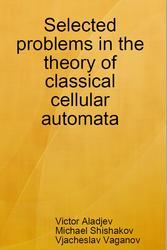# Selected problems in the theory of classical cellular automata, Aladjev V., Shishakov M., Vaganov V., 2018

Selected problems in the theory of classical cellular automata, Aladjev V., Shishakov M., Vaganov V., 2018.

In the book we present certain results of the work we have done in the theory of Classical Cellular Automata (CA). At present, these results form an essential constituent of the CA problematics. In particular, we have studied such problems as the nonconstructability problem in the CA, the decomposition problem of global transition functions in the CA, extremal constructive possibilities, the parallel formal grammars and languages defined by CA, complexity of finite configurations and global transition functions in the CA, simulation problem in classical CA, etc. At present, the CA problematics is a rather well developed independent field of the mathematical cybernetics that has a rather considerable field of various appendices. In addition, with the equal right the CA problematics can be considered as a component of such fields as discrete parallel dynamical systems, discrete mathematics, cybernetics, complex systems and some others. In our viewpoint, the book will represent an indubitable interest for students, postgraduates and persons working for doctor's degree of the appropriate faculties of universities, above all, of naturally scientific level along with teachers in subjects such as mathematical and physical modelling, discrete mathematics, automata theory, computer science, cybernetics, theoretical biology, computer technique, and a lot of others. In recent years, the classical CA models are one of the most promising simulating environments for various highly parallel discrete processes, objects and phenomena admitting reversible dynamics, that is enough important from a physical point of view, in the first place.The basic concepts of classical cellular automata (classical CA models).
In conformity with afore-said, the cellular automata (CA) at all generality present highly formalized models of some abstract Universes developing by simple rules and consisting of rather simple identical elements. The CA–universes of such kind develop according to local and everywhere identical rules of interaction of the elements forming them (laws). In this context we can consider CA–models as a certain analogue of a physical concept of «field». The space of CA–universe represents a regular lattice whose each cell presents a certain identical element (elementary particle, finite automaton, element) that receives a finite number of states.

Contents.
Introduction.
Chapter 1.The basic concepts of classical cellular automata.
Chapter 2.Nonconstructability problem in the classical cellular automata (classical CA models).
Chapter 3.Extremal constructive opportunities of the classical cellular automata.
Chapter 4.The complexity problem of finite configurations in the classical CA models.
Chapter 5.Parallel formal grammars and languages determined by the classical cellular automata (CA models).
Chapter 6.The modelling problem in the classical cellular automata (CA) along with the related questions.
Chapter 7.The decomposition problem of global transition functions in the classical CA models.
Chapter 8.Certain applied aspects of the CA problematics.
Conclusion.

Бесплатно скачать электронную книгу в удобном формате, смотреть и читать:
Скачать книгу Selected problems in the theory of classical cellular automata, Aladjev V., Shishakov M., Vaganov V., 2018 - fileskachat.com, быстрое и бесплатное скачивание.

Скачать zip
Ниже можно купить эту книгу по лучшей цене со скидкой с доставкой по всей России.Купить эту книгу

Скачать - zip,pdf - Яндекс.Диск.
Дата публикации:

Теги: :: :: :: :: :: :: :: :: ::